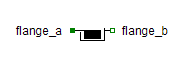Multiphase Quasi-RMS Current Sensor - MapleSim Help

Current Quasi-RMS Sensor

Multiphase quasi-rms current sensorDescription The Current Quasi-RMS Sensor component measures the continuous quasi-RMS value of multiphase current. If the current waveform deviates from a sine curve, the output of the sensor will not be exactly the average RMS value.Equations ${I}_{\mathrm{rms}}=\sqrt{\frac{1}{m}\sum _{k=1}^{m}{i}_{k}^{2}}$ $i={i}_{p}=-{i}_{n}$ $v={v}_{p}-{v}_{n}=0$Connections

 Name Description Modelica ID ${\mathrm{plug}}_{p}$ Positive $m$-phase plug plug_p ${\mathrm{plug}}_{n}$ Negative $m$-phase plug plug_n $I$ Real output; quasi-RMS of current in $A$ IParameters

 Name Default Units Description Modelica ID $m$ $3$ Number of phases mModelica Standard Library The component described in this topic is from the Modelica Standard Library. To view the original documentation, which includes author and copyright information, click here.